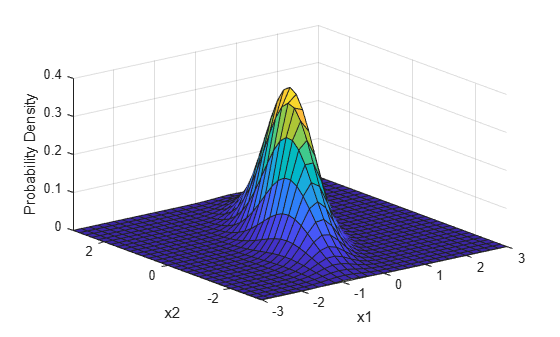## Multivariate Normal Distribution

### Overview

The multivariate normal distribution is a generalization of the univariate normal distribution to two or more variables. It is a distribution for random vectors of correlated variables, where each vector element has a univariate normal distribution. In the simplest case, no correlation exists among variables, and elements of the vectors are independent univariate normal random variables.

Because it is easy to work with, the multivariate normal distribution is often used as a model for multivariate data.

Statistics and Machine Learning Toolbox™ provides several functionalities related to the multivariate normal distribution.

### Parameters

The multivariate normal distribution uses the parameters in this table.

ParameterDescriptionUnivariate Normal Analogue
μMean vectorMean μ (scalar)
ΣCovariance matrix — Diagonal elements contain the variances for each variable, and off-diagonal elements contain the covariances between variablesVariance σ2 (scalar)

Note that in the one-dimensional case, Σ is the variance, not the standard deviation. For more information on the parameters of the univariate normal distribution, see Parameters.

### Probability Density Function

The probability density function (pdf) of the d-dimensional multivariate normal distribution is

where x and μ are 1-by-d vectors and Σ is a d-by-d symmetric, positive definite matrix.

Note that Statistics and Machine Learning Toolbox:

• Supports singular Σ for random vector generation only. The pdf cannot be written in the same form when Σ is singular.

• Uses x and μ oriented as row vectors rather than column vectors.

For an example, see Bivariate Normal Distribution pdf.

### Cumulative Distribution Function

The multivariate normal cumulative distribution function (cdf) evaluated at x is defined as the probability that a random vector v, distributed as multivariate normal, lies within the semi-infinite rectangle with upper limits defined by x,

`$\mathrm{Pr}\left\{v\left(1\right)\le x\left(1\right),v\left(2\right)\le x\left(2\right),...,v\left(d\right)\le x\left(d\right)\right\}.$`

Although the multivariate normal cdf has no closed form, `mvncdf` can compute cdf values numerically.

For an example, see Bivariate Normal Distribution cdf.

### Examples

#### Bivariate Normal Distribution pdf

Compute and plot the pdf of a bivariate normal distribution with parameters `mu = [0 0]` and `Sigma = [0.25 0.3; 0.3 1]`.

Define the parameters `mu` and `Sigma`.

```mu = [0 0]; Sigma = [0.25 0.3; 0.3 1];```

Create a grid of evenly spaced points in two-dimensional space.

```x1 = -3:0.2:3; x2 = -3:0.2:3; [X1,X2] = meshgrid(x1,x2); X = [X1(:) X2(:)];```

Evaluate the pdf of the normal distribution at the grid points.

```y = mvnpdf(X,mu,Sigma); y = reshape(y,length(x2),length(x1));```

Plot the pdf values.

```surf(x1,x2,y) axis([-3 3 -3 3 0 0.4]) xlabel('x1') ylabel('x2') zlabel('Probability Density')```#### Bivariate Normal Distribution cdf

Compute and plot the cdf of a bivariate normal distribution.

Define the mean vector `mu` and the covariance matrix `Sigma`.

```mu = [1 -1]; Sigma = [.9 .4; .4 .3];```

Create a grid of 625 evenly spaced points in two-dimensional space.

```[X1,X2] = meshgrid(linspace(-1,3,25)',linspace(-3,1,25)'); X = [X1(:) X2(:)];```

Evaluate the cdf of the normal distribution at the grid points.

`p = mvncdf(X,mu,Sigma);`

Plot the cdf values.

```Z = reshape(p,25,25); surf(X1,X2,Z)```#### Probability over Rectangular Region

Compute the probability over the unit square of a bivariate normal distribution, and create a contour plot of the results.

Define the bivariate normal distribution parameters `mu` and `Sigma`.

```mu = [0 0]; Sigma = [0.25 0.3; 0.3 1];```

Compute the probability over the unit square.

`p = mvncdf([0 0],[1 1],mu,Sigma)`
```p = 0.2097 ```

To visualize the result, first create a grid of evenly spaced points in two-dimensional space.

```x1 = -3:.2:3; x2 = -3:.2:3; [X1,X2] = meshgrid(x1,x2); X = [X1(:) X2(:)];```

Then, evaluate the pdf of the normal distribution at the grid points.

```y = mvnpdf(X,mu,Sigma); y = reshape(y,length(x2),length(x1));```

Finally, create a contour plot of the multivariate normal distribution that includes the unit square.

```contour(x1,x2,y,[0.0001 0.001 0.01 0.05 0.15 0.25 0.35]) xlabel('x') ylabel('y') line([0 0 1 1 0],[1 0 0 1 1],'Linestyle','--','Color','k')```Computing a multivariate cumulative probability requires significantly more work than computing a univariate probability. By default, the `mvncdf` function computes values to less than full machine precision, and returns an estimate of the error as an optional second output. View the error estimate in this case.

`[p,err] = mvncdf([0 0],[1 1],mu,Sigma)`
```p = 0.2097 ```
```err = 1.0000e-08 ```

 Kotz, S., N. Balakrishnan, and N. L. Johnson. Continuous Multivariate Distributions: Volume 1: Models and Applications. 2nd ed. New York: John Wiley & Sons, Inc., 2000.